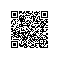# n皇后问题

n皇后

1. 路径：已经做出的选择
2. 选择列表：当前可以做的选择
3. 结束条件：到达决策树底层，无法再做选择的条件（路径==选择列表长度）

result = []

def backtrack(路径, 选择列表):

if 满足结束条件:

return

for 选择 in 选择列表:

做选择

backtrack(路径, 选择列表)

撤销选择

#include<cstdio>

#include<cstdlib>

#include<iostream>

using namespace std;

const int N=20;

int counter,n;//计数器：记录n皇后一共有几个解

int a[N];//表示第i行上的皇后放在a[i]列上；例a=7，表示第3行的皇后放在第7列

//检查第x行的皇后能否放在第y列

bool check(int x,int y){

//枚举前面x行

for(int i=1;i<x;i++){

if(a[i]==y) return false;//第i行已经存在皇后位于第y列

if(i+a[i]==x+y) return false;//右倾对角线上的点(x,y)，x+y都相等

if(i-a[i]==x-y) return false;//左倾对角线上的点(x,y),x-y都相等

}

return true;

}

void dfs(int row){//解决第row行皇后放在哪里

//到达边界

if(row==n+1){

//已经解决n行，产生了一组解

counter++;

return;

}

//未到达边界，则当前行的皇后有n种可能的放置

for(int i=1;i<=n;i++){

//对当前位置i进行check，能否放置

if(check(row,i)){

a[row]=i;//放置当前行皇后

dfs(row+1);//解决下一行的皇后位置，当其递归出时，说明已经产生了一组解

a[row]=0;//回收当前列皇后，准备将其放在下一列

}

}

}

int main(){

cin >> n;

dfs(1);

cout << counter;

return 0;

}

## 图着色问题

1. 无相连通图G
2. m种不同的颜色
3. G中每个顶点都要着色，且每条边的两个顶点颜色不同
4. 求共有多少种着色方法

//n:点个数

//c:邻接矩阵 c[i][j]=1表示i与j相连，=0表示不相连

//m:颜色数

//所有数组下标从1开始

void GraphColor(int n,int c[][],int m){

for(int i=1;i<=n;i++)//将数组color[n]初始化为0，表示顶点i尚未着色

color[i]=0;

k=1;

while(k>=1){

}

}使用钉钉扫一扫加入圈子
+ 订阅